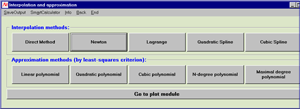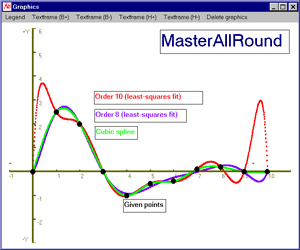# MasterAllRound: the PC calculator

## Interpolation and approximationEngineers and scientists are often faced with the problem of finding a function that matches a set of given data values. This problem is encountered, for example, when trying to fit a curve to a set of experimental values.

MasterAllRound offers selected interpolation methods and approximation functions, thus enabling you to solve your problem of constructing a function from a number of pairs of data values quickly and without pain.

#### Interpolation

The interpolating function exactly fits a given set of data values and represents the data between these as adequately as possible. The following interpolation methods are incorporated in MasterAllRound:• Direct polynomial interpolation
• Newton's divided difference interpolation formula
• Lagrange's polynomial
• Cubic spline

#### Approximation

For approximation, the polynomial function interpolates the data points as closely as possible (but maybe not exactly). The following least squares fit solutions are incorporated in MasterAllRound:
• Linear polynomial